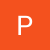# Build A Handwritten Digit Classifier on Azure Notebook using Pytorch

Source: Deep Learning on Medium

### What is Azure Notebook?

Azure Notebooks is a free service for anyone to develop and run code in their browser using Jupyter. Jupyter is an open source project that enables combing markdown prose, executable code, and graphics onto a single canvas. Azure Notebooks currently supports Python 2, Python 3, R, F# and their popular packages. All the developed code and used data will be stored on the cloud.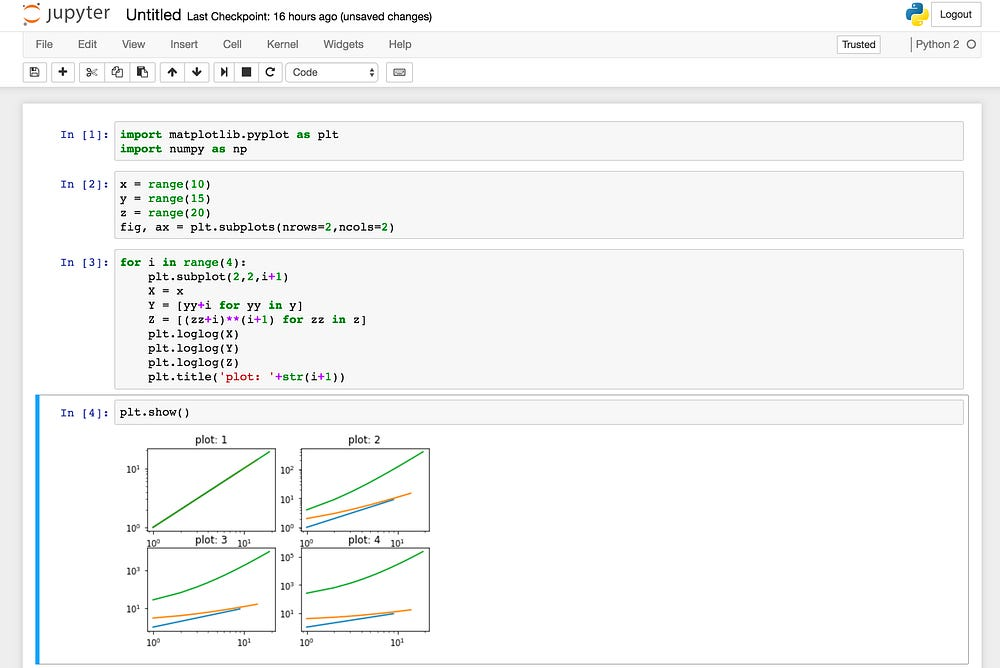Figure: The user interface of Jupyter Notebook.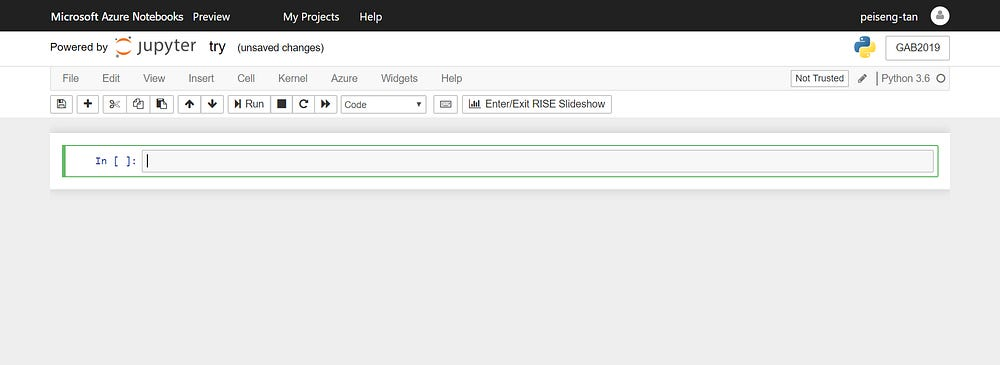Figure: The user interface of Azure Notebook.

### What is Pytorch?

PyTorch is an open-source deep learning library for Python, based on Torch, used for applications such as natural language processing, image recognition, image classification, text processing, etc. It is primarily developed by Facebook’s artificial-intelligence research group. PyTorch provides two high-level features:
— Tensor computation (like NumPy) with strong GPU acceleration
— Deep neural networks built on a tape-based autodiff system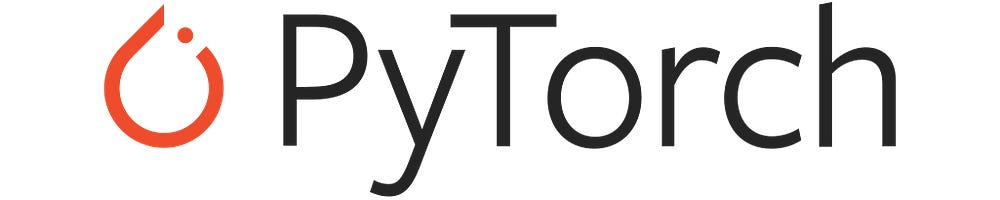Figure: The deep learning library that will be used for task later.

### The Origin of MNIST Handwritten Digit Dataset

The MNIST Handwritten Digit Dataset is contributed by Yann LeCun, Corinna Cortes and Christopher Burges to train a machine learning model to solve the issue of handwritten digit classification. Each image is a 28 by 28 pixel square (784 pixels total). This dataset contains 60,000 training images and 10,000 testing images in total for 10 different digits (0 to 10).

### Let’s Start To Build A Handwritten Digit Classifier

#### Step 1: Log in to Microsoft Azure Notebook:

Go to https://notebooks.azure.com/, login with your credential. After you successfully login, the screen will be automatically jumped to the figure as shown below.

Click on “My Projects” on the task-bar. Then, create a new project by pressing the button “New Project”.

Fill in the project name. After that, click the button “Create”.

A new folder for this project is created.

#### Step 2: Create two folder named “train” and “test”

Click on the button “+”, choose “Folder”.

Name the folder with “train”. Then, create another folder named “test” by using the same approach.

Two folders named “train” and “test” are ready now.

#### Step 3: Create new notebook named “demo”

Click on the button “+”, choose “Notebook”.

Fill in the Notebook Name as “Demo”. Choose “Python 3.6” and click button “New”.

A new notebook is created now for this project.

#### Step 4: Import the required libraries

1. Numpy — A fundamental package for scientific computing with Python. It contains a powerful N-dimensional array object, sophisticated (broadcasting) functions, tools for integrating C/C++ and Fortran code and useful linear algebra, Fourier transform, and random number capabilities.
2. Matplotlib — A Python 2D plotting library which produces publication quality figures in a variety of hard copy formats and interactive environments across platforms.
3. Torch — An open-source machine learning library, a scientific computing framework, and a script language based on the Lua programming language.
4. Torchvision — A package consists of popular datasets, model architectures, and common image transformations for computer vision.
5. Time — A module provides various time-related functions.
`import numpy as npimport matplotlib.pyplot as pltimport torchfrom torch import nn, optimimport torchvisionfrom torchvision import datasets, transformsfrom time import time`

#### Step 5: Define the pre-processing methods

Image transformations are applied onto the images so they can be fed into the model later for training or testing and improve the model accuracy.

1. transforms.RandomRotation(10) — Rotate the image in the range of [-10, 10] degrees randomly.
2. transforms.ToTensor() — Convert theimage to tensor.
3. transforms.Normalize((0.5,), (0.5,)) — Normalize the tensor image with mean and standard deviation. In this case, mean=0.5 and std=0.5.
`transform = transforms.Compose([transforms.RandomRotation(10),  transforms.ToTensor(), transforms.Normalize((0.5,), (0.5,)) ])`

#### Step 6: Preparation of training and testing dataset and dataloader

The operations of these codes are:

1. Download the MNIST handwritten digit recognition dataset into the “train” and “test” folders.
2. Generate the train and test datasets by inputting the downloaded images and transforming them based on defined pre-processing methods in the previous step.
3. Define the train and test loaders by setting 64 images will be fed into the model in each epoch randomly for training and non-randomly for testing.
`trainset = datasets.MNIST('train', download=True, train=True, transform=transform)testset = datasets.MNIST('test', download=True, train=False, transform=transform)trainloader = torch.utils.data.DataLoader(trainset, batch_size=64, shuffle=True)testloader = torch.utils.data.DataLoader(testset, batch_size=64, shuffle=False)`

#### Step 7: Data Visualization

The images can be reconstructed and plotted by loading one batch of images.

`dataiter = iter(trainloader)images, labels = dataiter.next()`
`print(images.shape)print(labels.shape)`

As you can see, the shape of the image is (64, 1, 28, 28). For each batch except the last batch, there are 64 grey-scale images. Each image contains 1 channel (grey-scale), 28 pixels of width and 28 pixels of height.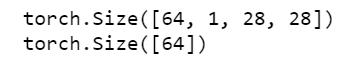Figure: Output of Azure Notebook after the codes above are executed.

Plot the images with Matplotlib library.

`figure = plt.figure()num_of_images = 60for index in range(1, num_of_images + 1): plt.subplot(6, 10, index) plt.axis('off') plt.imshow(images[index].numpy().squeeze(), cmap='gray_r')`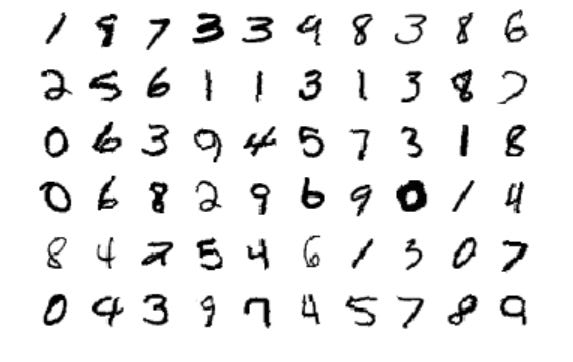Figure: Output of Azure Notebook after the codes above are executed.

#### Step 8: Define the network architecture

Define the network architecture for the model to train and test. For this task, there are only two hidden layers are required to perform the classification. These two hidden layers are considered as the fully connected layer. There is no any convolution and max-pooling layer in this model.

`input_size = 784hidden_sizes = [128, 64]output_size = 10`
`model = nn.Sequential(nn.Linear(input_size, hidden_sizes), nn.ReLU(), nn.Linear(hidden_sizes, hidden_sizes), nn.ReLU(), nn.Linear(hidden_sizes, output_size), nn.LogSoftmax(dim=1))print(model)`

#### Step 9: Define the criterion or loss function and optimizer

Negative Log Likelihood (NLL) Loss and Stochastic Gradient Descent (SGD) are chosen to be used as the criterion function and optimizer for this model respectively. You are welcome to try with other criterion functions and optimizes to get the optimum result. The value of learning rate (lr) and momentum are the hyper-parameters that you can play with as well.

`criterion = nn.NLLLoss()optimizer = optim.SGD(model.parameters(), lr=0.003, momentum=0.9)`

#### Step 10: Train the model

The operations of these codes are:

1. Record the start time of the training.
2. Set the training epoch to 20.
3. For each epoch, reset the running loss to 0, train the model then print the running loss after each epoch.
4. Record the end time of the training. Display the duration by using the end training time minus start training time.
`time0 = time()epochs = 20for e in range(epochs): running_loss = 0 for images, labels in trainloader: # Flatten images into a long vectors images = images.view(images.shape, -1) # Remove the accumulated gradient optimizer.zero_grad() # Feed-forward propagation output = model(images) # Compute the loss loss = criterion(output, labels) # Back-forward propagation  loss.backward() # Update the weight optimizer.step() # Record the loss to get total loss later running_loss += loss.item() print("Epoch {} - Training loss: {}".format(e, running_loss/len(trainloader)))print("\nTraining Time (in minutes) =",(time()-time0)/60)`

The lost of the model after 20 epochs of training is 0.0623. The time taken is 28.04 minutes.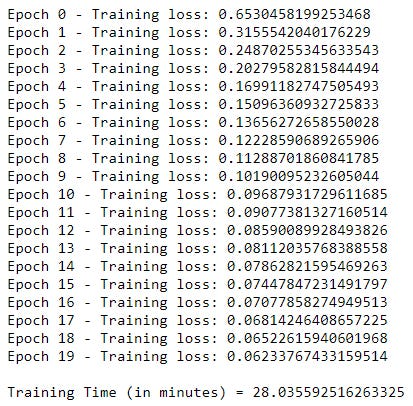Figure: Output of Azure Notebook after the codes above are executed.

#### Step 11: Test the model

The operations of these codes are:

1. Initialize the variables “correct_count” and “all_count” for accuracy calculation.
2. Load the test images, feed the images into the well-trained model and get the outputs.
3. Compare the outputs’ label with the original label. It will be counted as a correct prediction if output label is same with original label for each test image.
4. Display the accuracy score of the model. The accuracy of this model is 97.34%.
`correct_count, all_count = 0, 0for images, labels in testloader: for i in range(len(labels)): # Flatten images into a long vectors img = images[i].view(1, 784) with torch.no_grad(): logps = model(img) # Output of the network are log-probabilities, need to take exponential for probabilities ps = torch.exp(logps) probab = list(ps.numpy()) pred_label = probab.index(max(probab)) true_label = labels.numpy()[i] if(true_label == pred_label): correct_count += 1 all_count += 1`
`print("Number Of Images Tested =", all_count)print("\nModel Accuracy =", (correct_count/all_count))`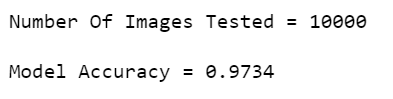Figure: The accuracy score of the trained model.

#### Step 12: Output Visualization

The predicted results can be shown together with the original images with the aid of Matplotlib library.

`images, labels = next(iter(testloader))fig=plt.figure(figsize=(10, 20))`
`for i in range (0, 10, 2): img1 = images[i].view(1, 784) img2 = images[i+1].view(1, 784) with torch.no_grad(): logps = model(img1) ps = torch.exp(logps) probab = list(ps.numpy()) fig.add_subplot(5, 2, i+1) plt.imshow(img1.resize_(1, 28, 28).numpy().squeeze()) plt.title("Predicted Digit = {}".format(probab.index(max(probab)))) logps = model(img2) ps = torch.exp(logps) probab = list(ps.numpy()) fig.add_subplot(5, 2, i+2) plt.imshow(img2.resize_(1, 28, 28).numpy().squeeze()) plt.title("Predicted Digit = {}".format(probab.index(max(probab))))`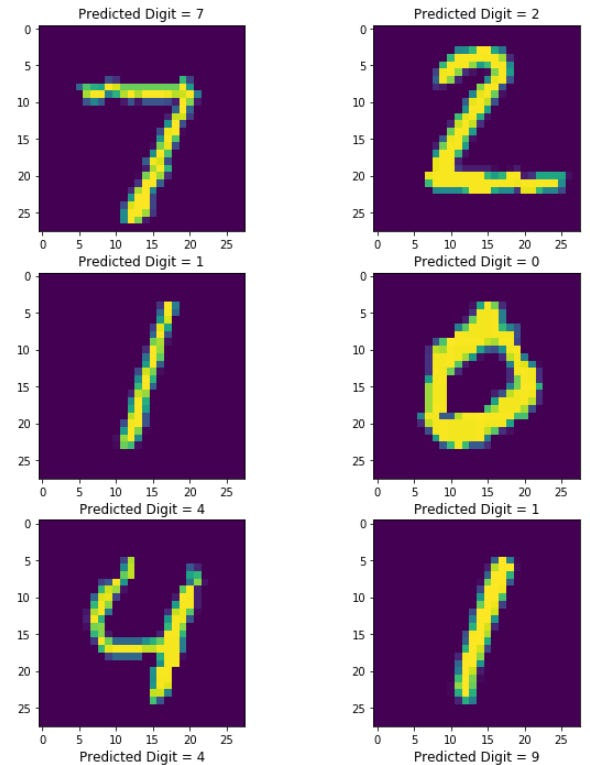Figure: Output of Azure Notebook after the codes above are executed.

#### Step 13: Save the model

The model is saved in the filename of “demo.model”.

`torch.save(model.state_dict(), "demo.model")`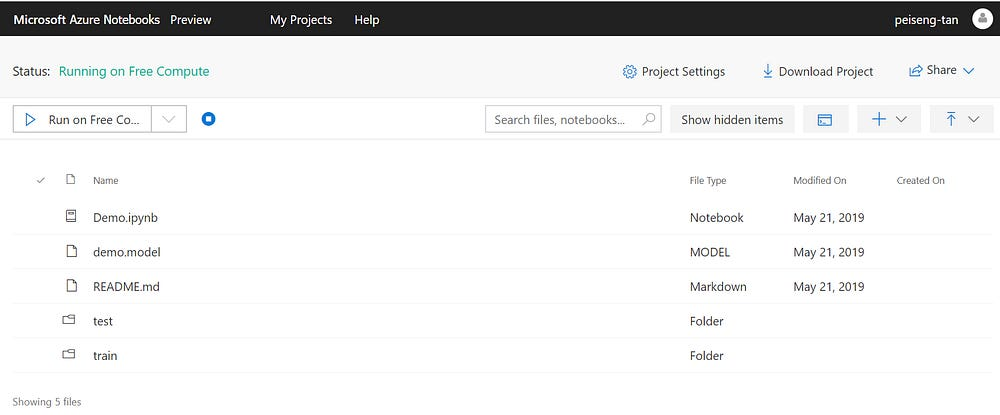Figure: The model is saved in the Azure Notebook.

### References

1. Help and Documentation of Microsoft Azure Notebook https://notebooks.azure.com/help/introduction
2. Wikipedia — Pytorch
https://en.wikipedia.org/wiki/PyTorch
3. The origin of dataset
http://yann.lecun.com/exdb/mnist/
4. Handwritten Digit Recognition Using PyTorch — Intro To Neural Networks
https://towardsdatascience.com/handwritten-digit-mnist-pytorch-977b5338e627
5. Pytorch Documentation
https://pytorch.org/docs/stable/index.html